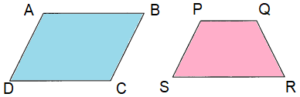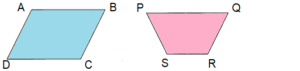# Math in Focus Grade 3 Chapter 18 Practice 1 Answer Key Classifying Polygons

This handy Math in Focus Grade 3 Workbook Answer Key Chapter 18 Practice 1 Classifying Polygons provides detailed solutions for the textbook questions.

## Math in Focus Grade 3 Chapter 18 Practice 1 Answer Key Classifying Polygons

Circle the polygons.

Question 1.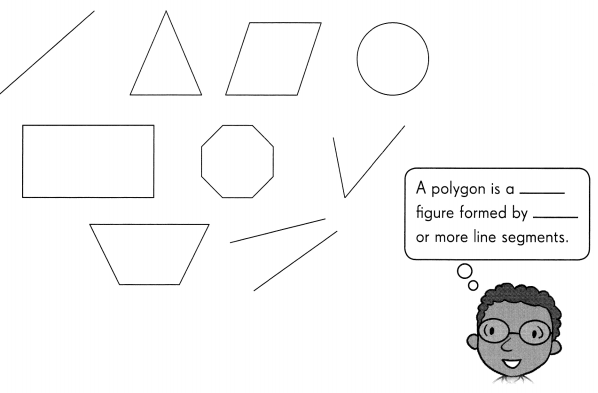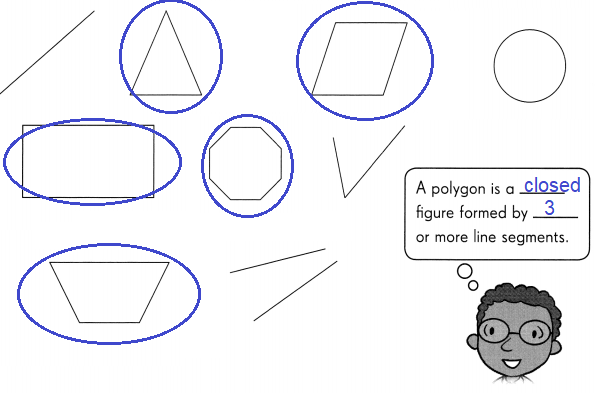Explanation:
The above figure contains 5 polygons.
A polygon is a closed two-dimensional shape that is formed by enclosing line segments.
A minimum of three line segments are required to make a polygon.

Mark the angles. Label the parts of each polygon.

Question 2.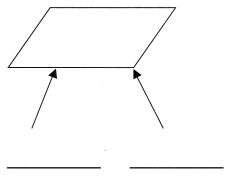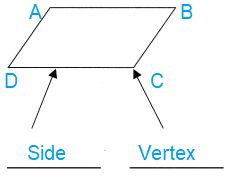Explanation:
Given figure is a polygon.
The line segments forming a polygon are called its sides.
Sides are AB, BC, CD  and DA .
The meeting point of a pair of sides is called its vertex.

Question 3.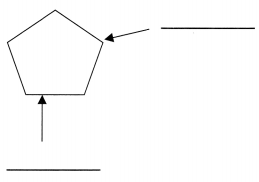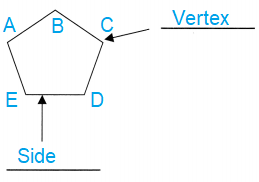Explanation:
Given picture is called a polygon.
The line segments forming a polygon are called its sides.
Sides are AB, BC, CD, DE and EA .
The meeting point of a pair of sides is called its vertex.

Identify each polygon.

Question 4.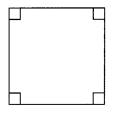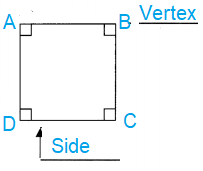Explanation:
Given figure is a polygon. Square in shape.
The line segments forming a polygon are called its sides.
Sides are AB, BC, CD  and DA .
The meeting point of a pair of sides is called its vertex.

Question 5.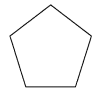Explanation:
Given picture is called a polygon. Pentagon in shape.
The line segments forming a polygon are called its sides.
Sides are AB, BC, CD, DE and EA .
The meeting point of a pair of sides is called its vertex.

Question 6.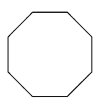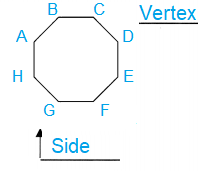Explanation:
Given picture is called a polygon. Octagon in shape.
The line segments forming a polygon are called its sides.
What are the sides of polygon ABCDEFGH
Sides are AB, BC, CD, DE , EF and FG, GH and HA .
The meeting point of a pair of sides is called its vertex.

Question 7.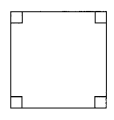Explanation:
Given figure is a polygon. Square in shape.
The line segments forming a polygon are called its sides.
Sides are AB, BC, CD  and DA .
The meeting point of a pair of sides is called its vertex.

Question 8.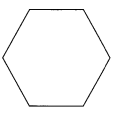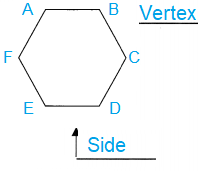Explanation:
Given picture is called a polygon. Hexagon in shape.
The line segments forming a polygon are called its sides.
What are the sides of polygon ABCDEF
Sides are AB, BC, CD, DE , EF and FA .
The meeting point of a pair of sides is called its vertex.

Question 9.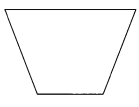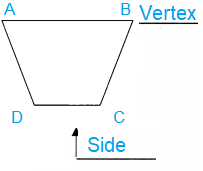Explanation:
Given figure is a polygon. Trapezoid in shape.
The line segments forming a polygon are called its sides.
Sides are AB, BC, CD  and DA .
The meeting point of a pair of sides is called its vertex.

Complete the table. Then answer the question.

Question 10.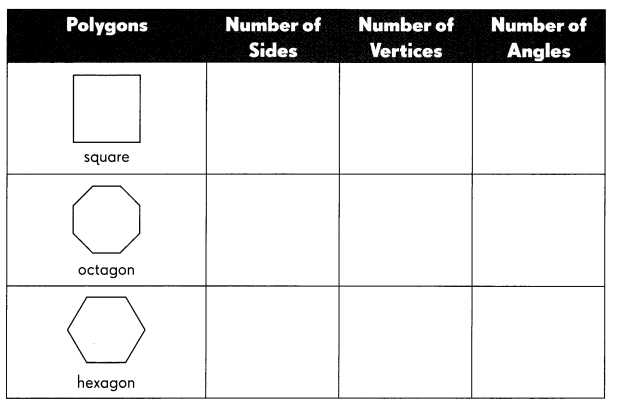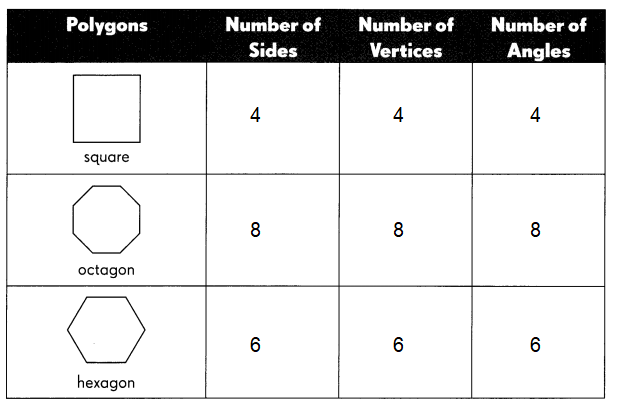Explanation:
A Square is a regular quadrilateral or polygon.
which has all the four sides of equal length, four vertices and,
four angles with right angle triangles.
Octagon is a polygon in geometry, which has 8 sides, 8 vertices and 8 angles.
That means the number of vertices is 8 and the number of edges is 8.
So, octagon is a 8-sided polygon and it is a two dimensional plane figure.
All the sides are joined with each other end-to-end to form a shape.
In geometry, a hexagon can be defined as a polygon with six sides.
The two-dimensional shape has 6 sides, 6 vertices and 6 angles.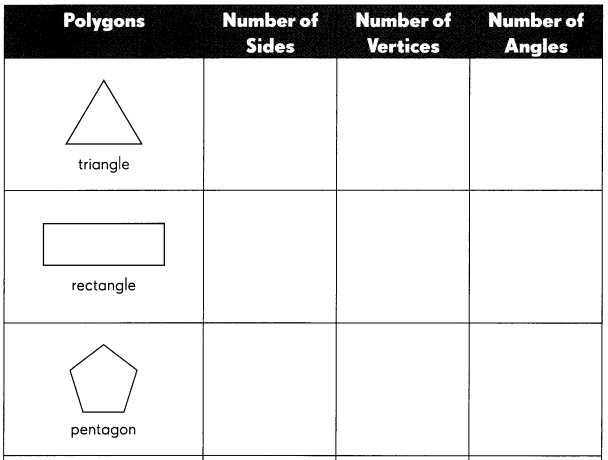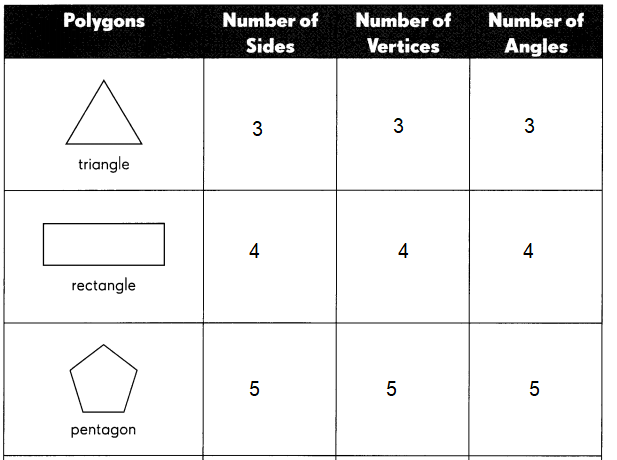Explanation:
The above Triangle is polygon.
A triangle has three sides, 3 vertices and
3 angles sum to 180 degrees.
The above figure rectangular polygon,
it has four sides, four vertices and four angles, with 90 degrees.
And the angles are right angle triangles.
A pentagon is a five-sided polygon with five straight sides,
five vertices and five interior angles that sum up to 540°540°.
A pentagon shape is a plane figure, or flat (two-dimensional) 5-sided geometric shape.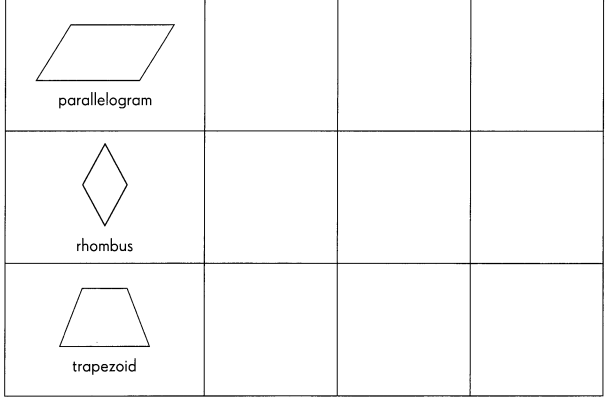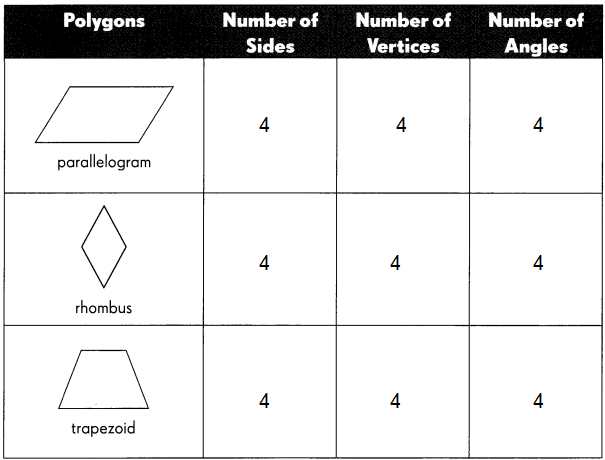Explanation:
Parallelogram is a four-sided closed figure with opposite sides are equal and opposites angles are equal.
A parallelogram has 4 sides, 4 vertices and 4 angles.
A rhombus is a quadrilateral whose four sides have the same length.
Every rhombus has two diagonals connecting pairs of opposite vertices, and two pairs of parallel sides.
Opposite angles of a rhombus have equal length.
A trapezoid is a quadrilateral with exactly one pair of parallel sides.
The sum of the interior angles of a trapezoid equals 360 degrees,
So, it has four angles and four vertices, also called corners.

Question 11.
Which figures have the same number of sides, vertices and angles?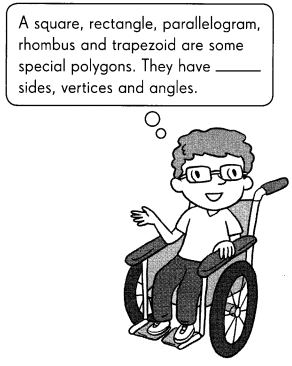All the above mentioned polygons have sides, vertices and angles.
Explanation:
Polygons are named according to the number of sides and angles they have.
The most familiar polygons are the triangle, the rectangle, and the square.
A regular polygon is one that has equal sides.
Some special polygons have equal sides, vertices and angles.

Are these statements correct? Write true or false.

Question 12.
A hexagon has seven sides and six angles. ____________
Explanation:
hexa means six
In geometry, a hexagon can be defined as a polygon with six sides.
The two-dimensional shape has 6 sides, 6 vertices and 6 angles.

Question 13.
All polygons have four sides. ____________
Explanation:
A plane figure that is described by a finite number of straight line segments connected to form a closed polygon.
Polygons are named according to the number of sides and angles they have.

Question 14.
All parallelograms, squares, and trapezoids have four angles. ____________
Explanation:
All parallelograms, squares, and trapezoids have four angles,
4 sides and 4 vertices as mentioned above.

Question 15.
An octagon has eight vertices and seven sides. ____________
Explanation:
Octagon is a polygon in geometry, which has 8 sides, 8 vertices and 8 angles.
That means the number of vertices is 8 and the number of edges is 8.
So, octagon is a 8-sided polygon and it is a two dimensional plane figure.
All the sides are joined with each other end-to-end to form a shape.

Question 16.
A pentagon has six angles. ____________
Explanation:
A pentagon is a five-sided polygon with five straight sides,
five vertices and five interior angles that sum up to 540°540°.
A pentagon shape is a plane figure, or flat (two-dimensional) 5-sided geometric shape.

Question 17.
A triangle has two vertices. ____________
Explanation:
A triangle has three sides, 3 vertices and
3 angles sum to 180 degrees.

Question 18.
A parallelogram can be separated into 4 triangles. ____________
Explanation:
Parallelogram is a four-sided closed figure with opposite sides are equal and opposites angles are equal.
A parallelogram has 4 sides, 4 vertices and 4 angles.

Question 19.
A rectangle has four right angles. ____________
Explanation:
A rectangle has four sides, four vertices and four angles, with 90 degrees.
And the angles are right angle triangles.

Cut out the tangram below and complete the table.

Question 20.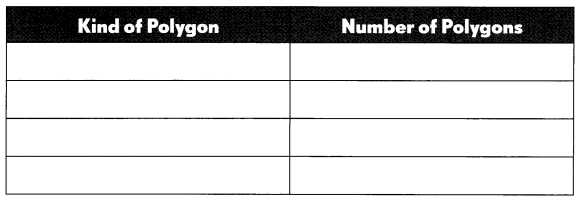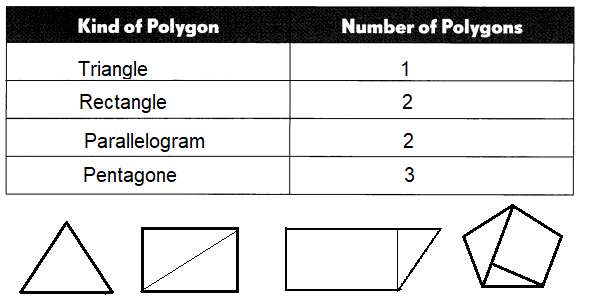Explanation:
We can write all polygons like square, triangle, rectangle and pentagon …. so on.
Polygons are named according to the number of sides and angles they have.
The most familiar polygons are the triangle, the rectangle, and the square.
A regular polygon is one that has equal sides.
Some special polygons have equal sides, vertices and angles.

Use at least 5 polygons to make a figure. Complete the table.

Question 21.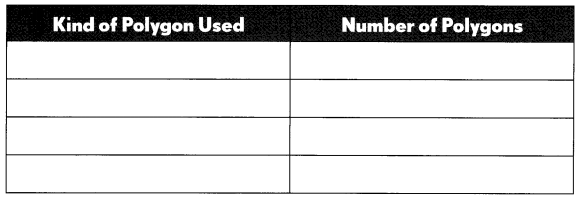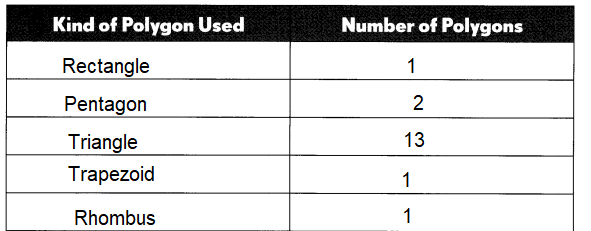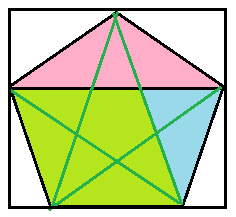Explanation:
All types of polygons be used.
here in the above figure we used 5 polygons
i) Pentagon
ii) Triangle
iii) Rhombus
iv) Trapezoid
v) Rectangle
As we know polygon is a two-dimensional geometric figure that has a finite number of sides and a closed figure.
Polygons are named according to the number of sides and angles they have.
A regular polygon is one that has equal sides.
Some special polygons have equal sides, vertices and angles.

Question 22.
Name the figure that you have made.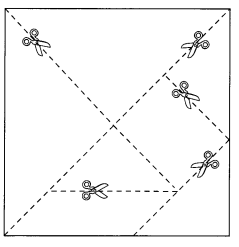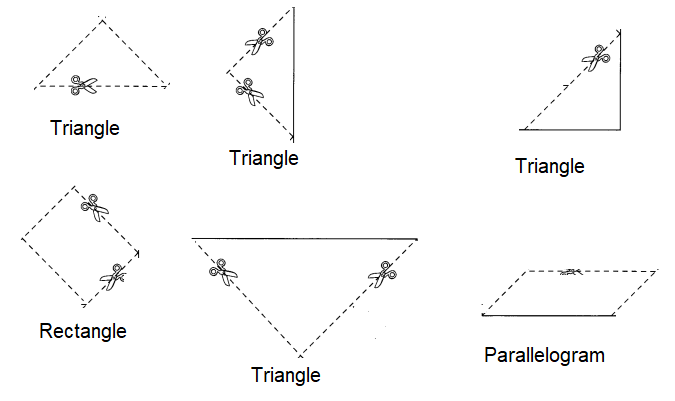Explanation:
From the above figure,
we can cut 4 triangles, 1 parallelogram and 1 rectangle.

Solve.

Question 23.
I am a polygon. I have 1 more angle than a rectangle has. What am I? ___________
Pentagon
Explanation:
A pentagon is a five-sided polygon with five straight sides,
five vertices and five interior angles that sum up to 540°540°.
A pentagon shape is a plane figure, or flat (two-dimensional) 5-sided geometric shape.

Question 24.
I am a polygon. I have 1 more side than a pentagon has. What am I? ___________
Hexagon
Explanation:
hexa means six
In geometry, a hexagon can be defined as a polygon with six sides.
The two-dimensional shape has 6 sides, 6 vertices and 6 angles.

Question 25.
I am a polygon. I have 1 more vertex than a triangle has. What am I? ___________
Rectangle
Explanation:
A rectangle has four sides, four vertices and four angles, with 90 degrees.
And the angles are right angle triangles.

Question 26.
Add one more polygon to the shape below to make it a hexagon.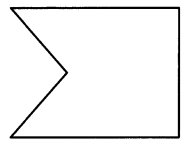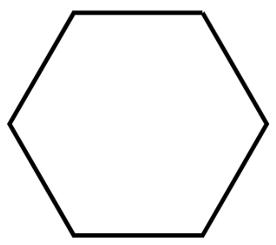Explanation:
The above drawn figure is Hexagon, hexa means six.
In geometry, a hexagon can be defined as a polygon with six sides.
The two-dimensional shape has 6 sides, 6 vertices and 6 angles.

Example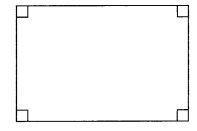This is a rectangle. A rectangle has 2 pairs of opposite sides that are parallel. Only the opposite sides of a rectangle need to be of equal length. All 4 angles of a rectangle are right angles.

Question 27.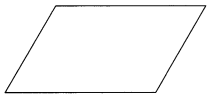This is a ____________________
This is a parallelogram.
Explanation:
A parallelogram has 2 pairs of opposite sides that are parallel.
Only the opposite sides of a rectangle need to be of equal length.
All 4 angles of a rectangle are right angles.

Question 28.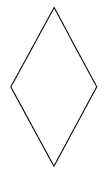This is a ____________________
This is a rhombus.
Explanation:
A rhombus has 2 pairs of opposite sides that are parallel.
Only the opposite sides of a rectangle need to be of equal length.
All 4 angles of a rectangle are right angles.

Question 29.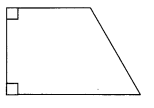This is a ____________________
This is a Trapezoid.
Explanation:
A trapezoid has 2 pairs of opposite sides that are parallel.
Only the opposite sides of a rectangle need to be of equal length.
All 4 angles of a rectangle are right angles.

Write P for a parallelogram, R for rhombus, or T for trapezoid on the shapes.

Question 30.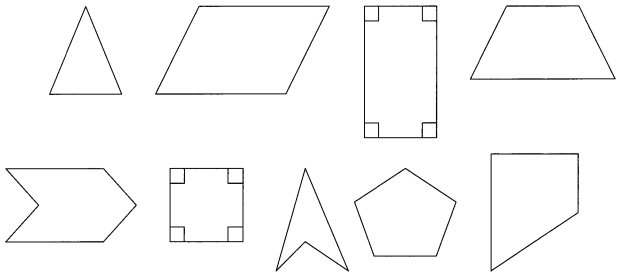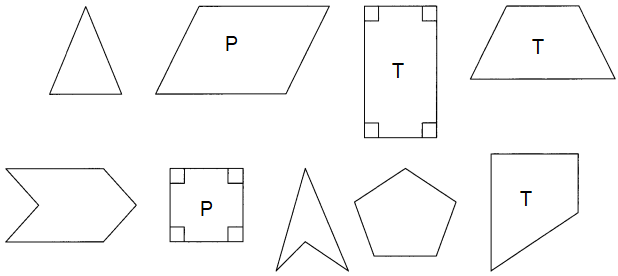Explanation:
As we all ready know that clearly about Parallelogram, Rhombus and Trapezoid in the above question no.27,
identify the Parallelogram, Rhombus and Trapezoid and mention with their initials.

Question 31.
How is a trapezoid different from a parallelogram?